﻿ RELAP5对低压自然循环系统的分析能力研究
«上一篇文章快速检索 高级检索

 哈尔滨工程大学学报2019, Vol. 40Issue (5): 920-925  DOI: 10.11990/jheu.2018030560

### 引用本文WANG Qiang, GAO Puzhen, WANG Zhongyi, et al. Analysis capability of RELAP5 in low-pressure natural circulation systems[J]. Journal of Harbin Engineering University, 2019, 40(5), 920-925. DOI: 10.11990/jheu.201803056.### 文章历史

RELAP5对低压自然循环系统的分析能力研究

Analysis capability of RELAP5 in low-pressure natural circulation systems
WANG Qiang , GAO Puzhen, WANG Zhongyi , CHEN Xianbing , HUANG Ying
Fundamental Science on Nuclear Safety and Simulation Technology Laboratory, Harbin Engineering University, Harbin 150001, China
Abstract: To investigate the analysis capability of RELAP5 in low-pressure natural circulation systems, a series of natural circulation experiments was performed under various conditions such as steady state, transient state and flow instability. Further, the sensitivity oflow-pressure natural circulation systems was discussed based on the reactor transient thermal-hydraulic RELAP5 program. The sensitivity analysis of space and time step was carried out, and the test criteria of time step sensitivity were provided. Subsequently, the ability of RELAP5 was evaluated by the simulation and experiments of flow instability under a type of flowing condition. The results indicate that the independence can be verified according to the Courant number for the two-phase natural circulation problem; further, appropriate model selection and parameter sensitivity verification can effectively improve the simulation accuracy for the complex dynamic flow instability observed in systems with high heat flux density.When these results are combined with the fast Fourier transform analysis result.It can be observed that the periodic phase change process occurring in the long straight riser is a non-linear load with composite dynamic flow instability.
Keywords: natural circulation    flow instability    RELAP5    transient character    low pressure    sensitivity analysis    Courant number    fast Fourier transform (FFT)

Hamidouche等和William等分别利用RELAP5程序对低压条件下的不稳定性起始点及相变模型进行了研究。然而，强迫循环高流速条件下得到的研究结果，不足以评估该程序对自然循环问题的分析能力。绝大多数的自然循环实验系统(如SIRIUS-N，CIRCUS等)都运行在中、低压条件下，两相自然循环在该条件下的模拟计算仍然是难点。程坤等通过对动量方程的修改，对RELAP进行了二次开发，有效提高了该程序对摇摆条件下瞬态问题的分析能力。Kozmenkov对低压自然循环下闪蒸引起的流动不稳定性进行了程序验证，数值计算结果与实验数据吻合较好，证明了RELAP5能够解决与闪蒸相关的不稳定性问题。

1 实验装置及实验过程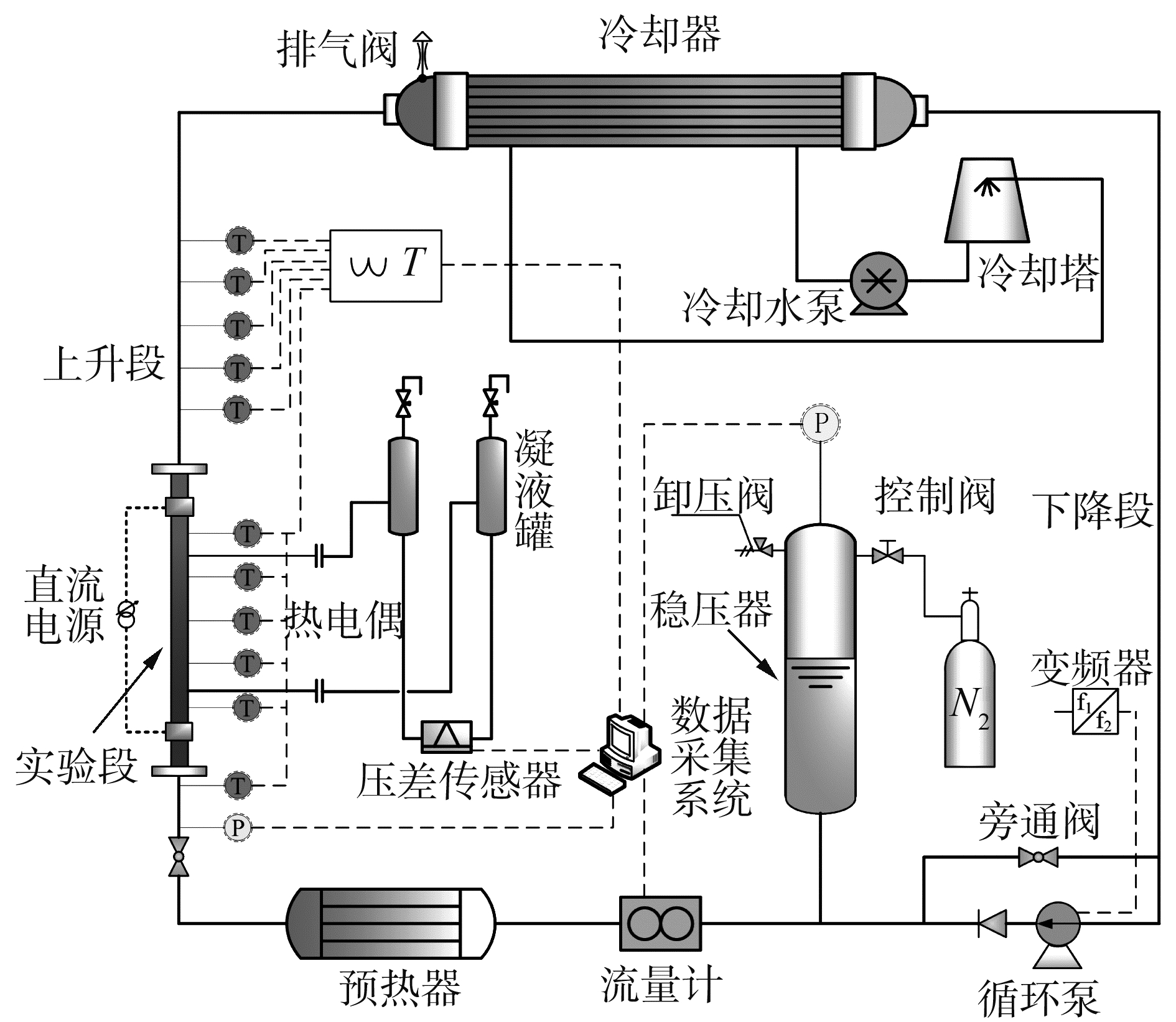Download: 图 1 实验装置示意 Fig. 1 Schematic of experimental apparatus

2 RELAP5计算模型简介 2.1 自然循环系统节点划分概述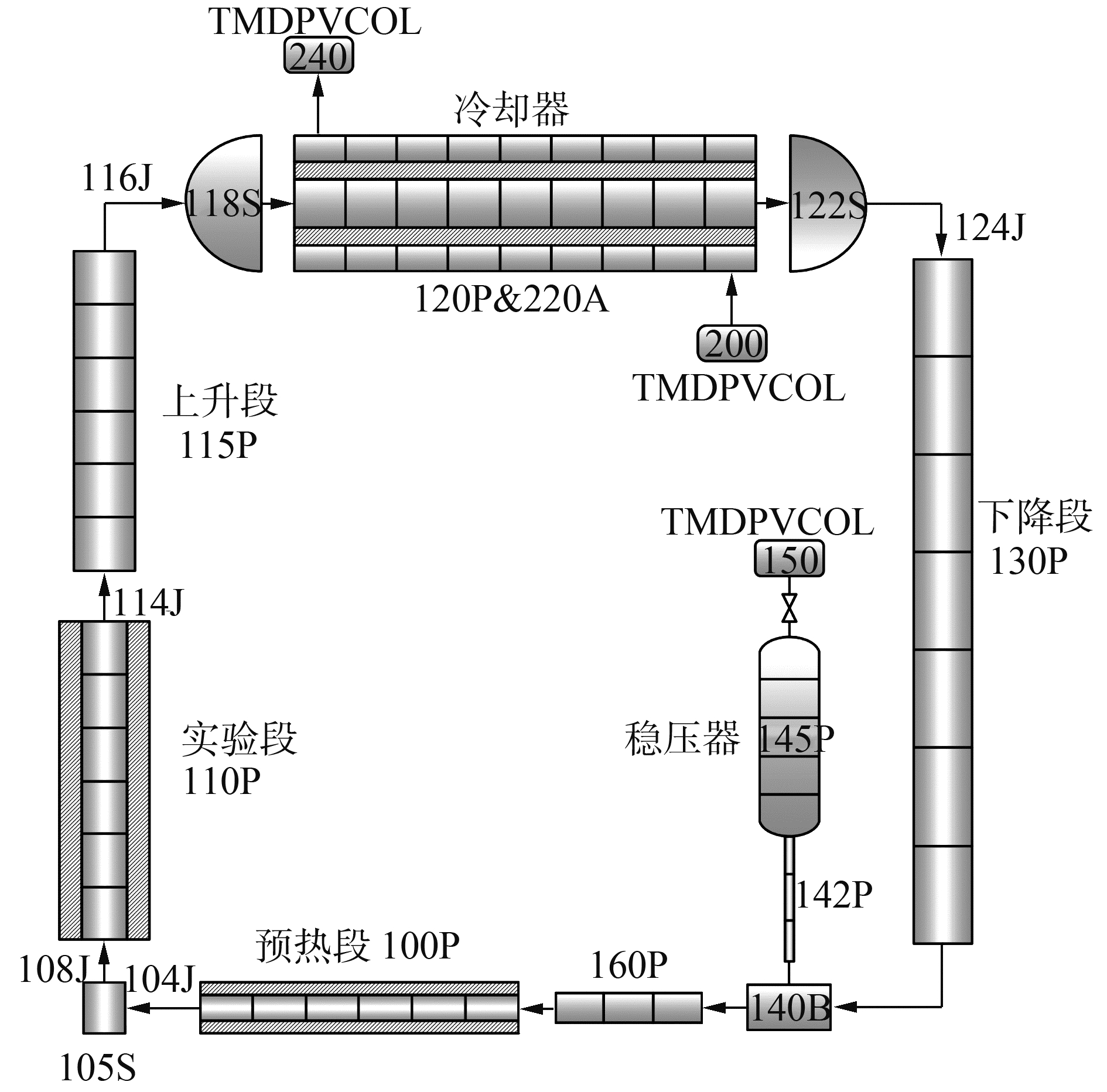Download: 图 2 自然循环系统RELAP5节点 Fig. 2 Layout of the RELAP5 nodalization for natural circulation system

2.2 模型选择

RELAP5/MOD3.3流体动力学模型采用一维、瞬态、两流体模型来解决两相混合物的流动问题。在数理模型的建立过程中，可以根据实际情况，利用自定义函数来修改RELAP5的模型。结合本实验系统，使用的主要模型为：

1) 壁面摩擦模型。

 ${\lambda _{\rm{L}}} = 64/Re, \;\;\;\;\;0 \le Re < 2200$ (1)
 $\begin{array}{*{20}{c}} {{\lambda _{{\rm{L, T}}}} = (3.75 - 8250/{\rm{Re}})\left( {{\lambda _{T, 3000}} - {\lambda _{{\rm{L}}, 2200}}} \right) + }\\ {{\lambda _{{\rm{L, 2200}}}}, \quad 2200 \le Re < 3000} \end{array}$ (2)

 $\begin{array}{l} \frac{1}{{\sqrt {{\lambda _{\rm{T}}}} }} = - 2\;{\rm{lg}}\left\{ {\frac{\varepsilon }{{3.7{D_{\rm{h}}}}} + \frac{{2.51}}{{Re}} \times } \right.\\ \;\;\;\;\;\;\;\;\;\left[ {1.14 - 2\;{\rm{lg}}\left( {\frac{\varepsilon }{{{D_{\rm{h}}}}} - \frac{{21.25}}{{R{e^{0.9}}}}} \right)} \right]\} \end{array}$ (3)

2) 两相传热模型。

 ${\mathit{\Gamma }_{{\rm{ig}}}} = - \frac{{{H_{{\rm{ig}}}}\left( {{T_s} - {T_{\rm{g}}}} \right) + {H_{{\rm{if}}}}\left( {{T_{\rm{s}}} - {T_{\rm{f}}}} \right)}}{{h_{\rm{g}}^s - {h_{\rm{f}}}}}, {\mathit{\Gamma }_{{\rm{ig}}}} \ge 0$ (4)

 ${\mathit{\Gamma }_{{\rm{ig}}}} = - \frac{{{H_{{\rm{ig}}}}\left( {{T_s} - {T_{\rm{g}}}} \right) + {H_{{\rm{if}}}}\left( {{T_{\rm{s}}} - {T_{\rm{f}}}} \right)}}{{{h_{\rm{g}}} - h_{\rm{f}}^s}}, {\mathit{\Gamma }_{{\rm{ig}}}} \ge 0$ (5)

 ${\mathit{\Gamma }_{{\rm{wg}}}} = \frac{{q{A_{\rm{w}}}}}{{V\left( {h_{\rm{g}}^s - {h_{\rm{f}}}} \right)}} \times \frac{{{\rm{min}}\left( {{h_{\rm{f}}}, h_{\rm{f}}^s} \right) - {h_{{\rm{er}}}}}}{{\left( {h_{\rm{s}}^f - {h_{{\rm{cr}}}}} \right)(1 + \varepsilon )}}$ (6)

3 参数敏感性分析 3.1 空间步长敏感性

 $\zeta = \frac{{\Delta L}}{{{D_h}}} \ge 0.5$ (7)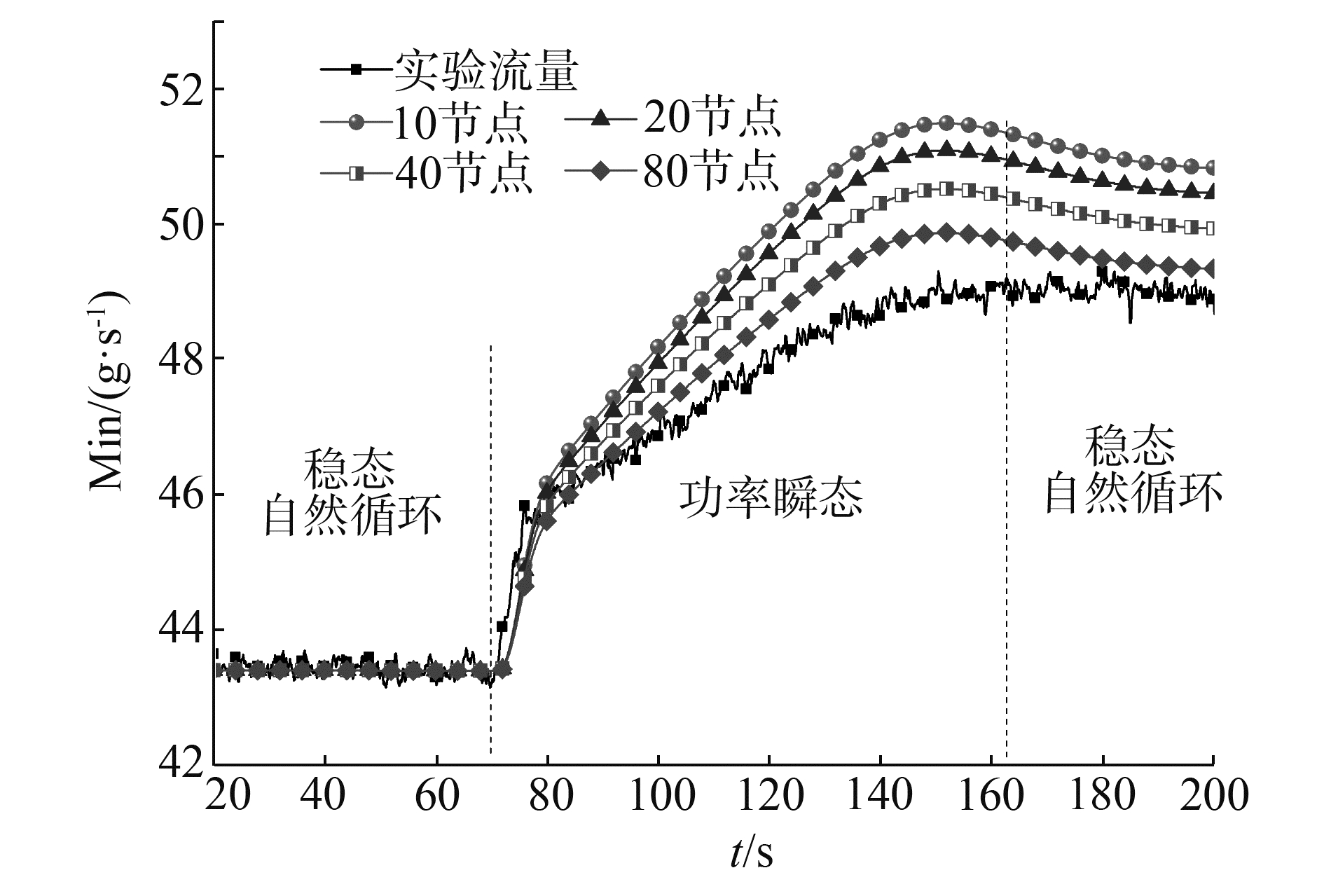Download: 图 3 节点划分对自然循环瞬态特性的影响 Fig. 3 The impact of nodalization on natural circulation transient character

3.2 时间步长敏感性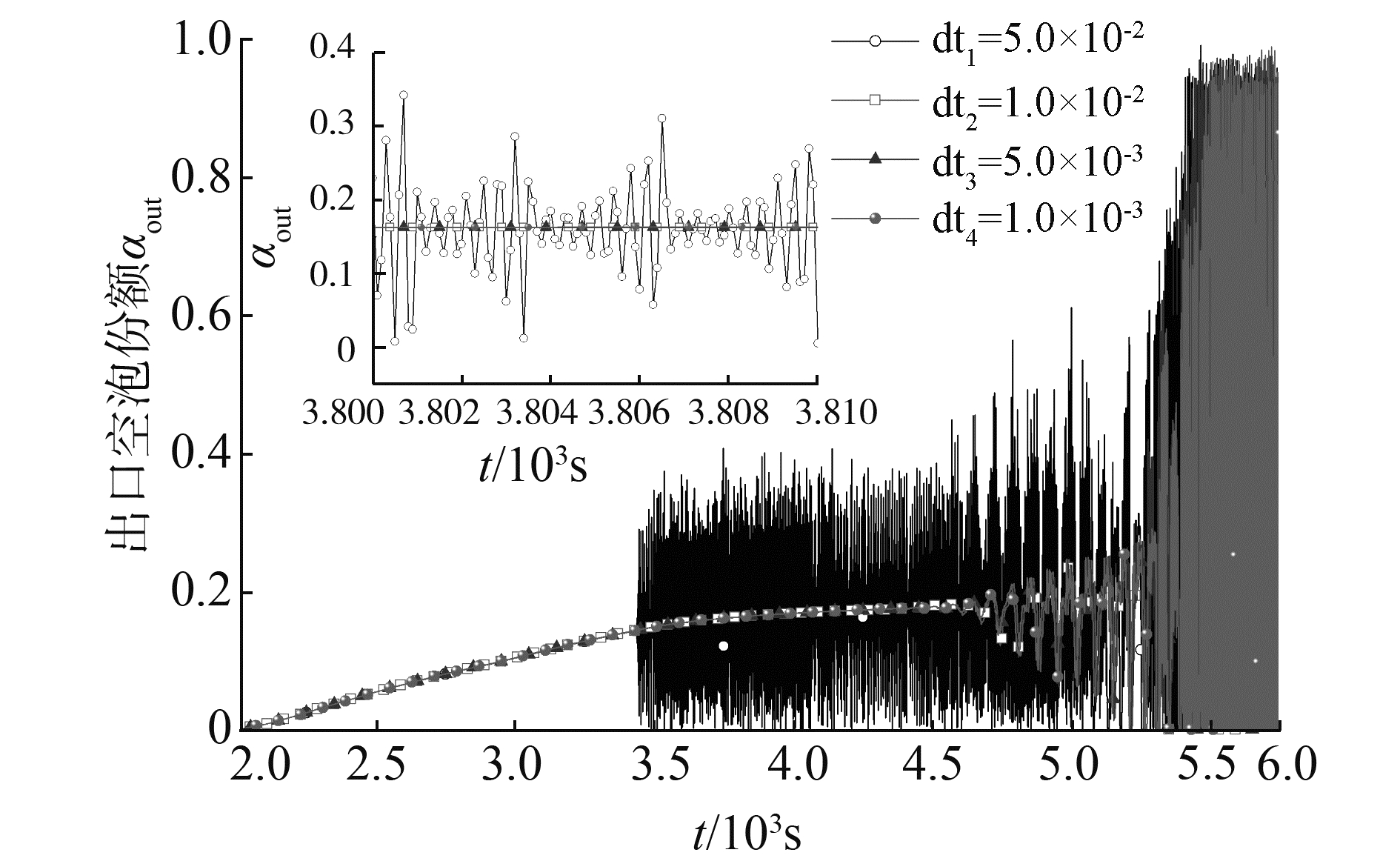Download: 图 4 时间步长对两相自然循环的影响 Fig. 4 The influence of time step on two-phase natural circulation

 $C_{i}=\frac{u \Delta t}{\Delta z}=\frac{\overline{M} \Delta t}{\rho_{i} A_{i} \Delta z}$ (8)表 1 不同库郎数时收敛性验证 Table 1 Validation of convergence for courant number

4 流动不稳定性分析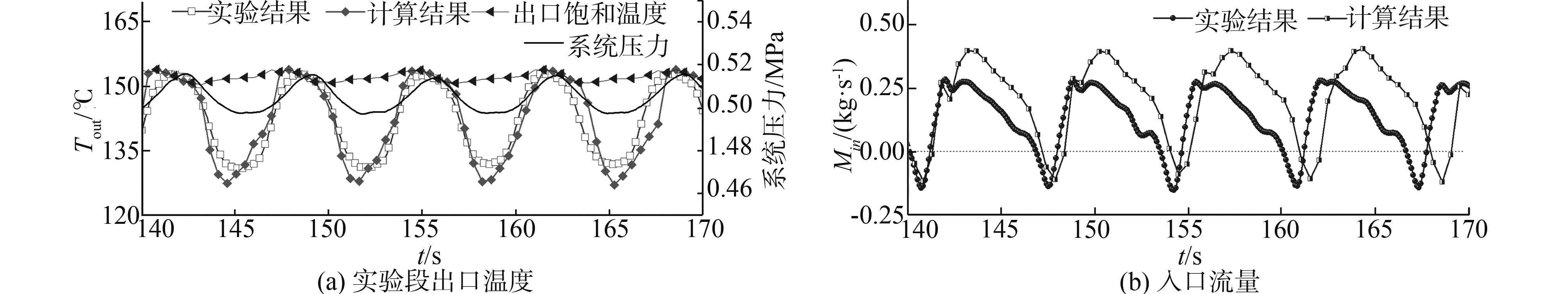Download: 图 5 实验结果与计算结果对比 Fig. 5 Comparison between experimental data and calculation results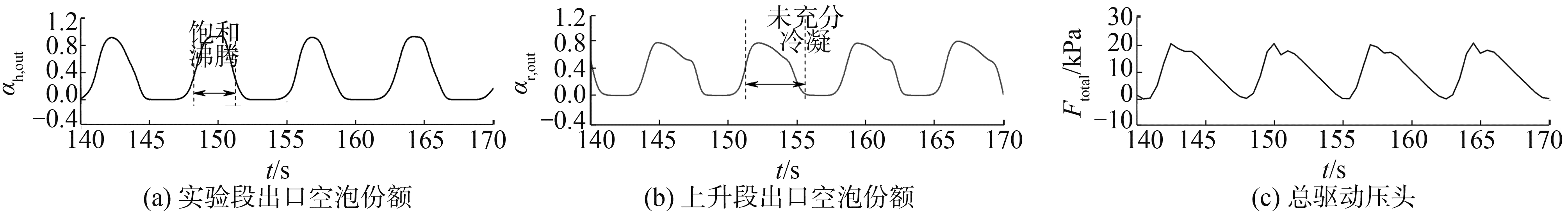Download: 图 6 流动不稳定期间主要参数变化规律 Fig. 6 Variation of main parameters during flow instability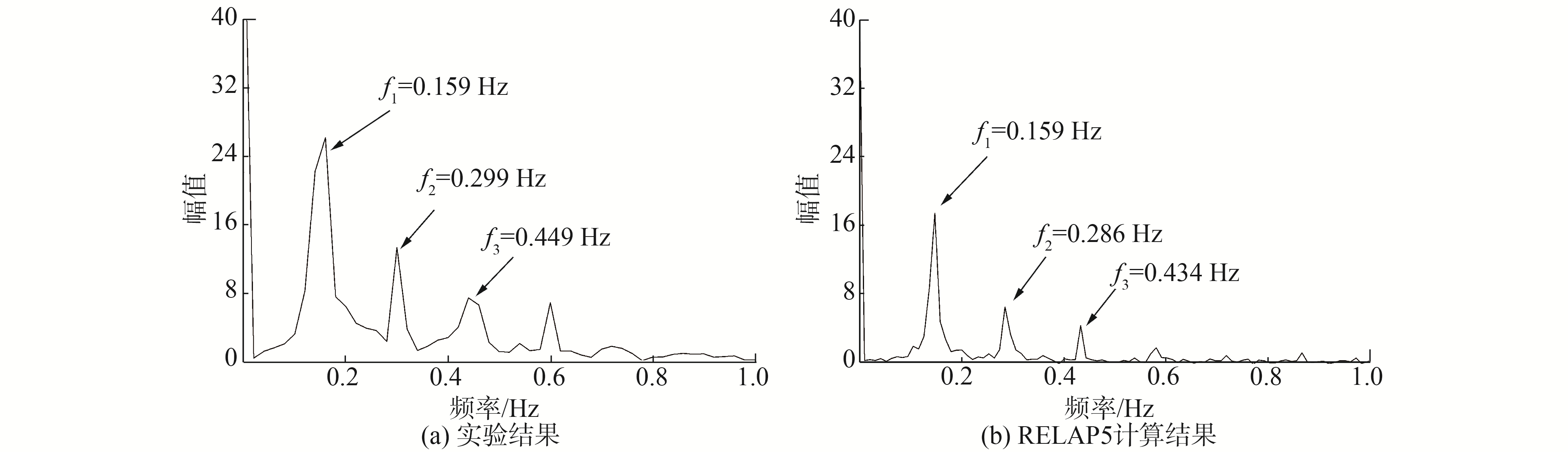Download: 图 7 复合动态流动不稳定性幅度谱图 Fig. 7 The amplitude spectrum of compound dynamic instability

5 结论

1) 针对低压自然循环实验系统，利用RELAP5建立了能够计算自然循环系统特性的数理模型。能够较准确地模拟自然循环系统的各瞬态特性。

2) 对低压两相自然循环问题，模化过程必须细致，尤其对于有相变存在的部件。两相自然循环瞬态过程对时间步长有一定的依赖性，仅对空间步长进行无关性检验，不能完全保证自然循环瞬态分析的正确性。无关性验证时应保证库郎数不大于1。

3) RELAP5能够实现对高热流密度下的自然循环流动不稳定现象的模拟，不稳定性起始点预测值偏低。

  徐济鋆. 沸腾传热和气液两相流[M]. 2版. 北京: 原子能出版社, 2001: 183-188. (0)  谭思超, 高璞珍, 苏光辉. 摇摆运动条件下自然循环流动的实验和理论研究[J]. 哈尔滨工程大学学报, 2007, 28(11): 1213-1217. TAN Sichao, GAO Puzhen, SU Guanghui. Experimental and theoretical study on natural circulation flow under rolling motion condition[J]. Journal of Harbin Engineering University, 2007, 28(11): 1213-1217. DOI:10.3969/j.issn.1006-7043.2007.11.005 (0)  Nuclear Safety Analysis Division. RELAP5/MOD3.3 code manual volume Ⅰ:code structure, system models, and solution methods[M]. US: Nuclear Regulatory Commission, 2001. (0)  HAMIDOUCHE T, BOUSBIA-SALAH A. RELAP5/3.2 assessment against low pressure onset of flow instability in parallel heated channels[J]. Annals of nuclear energy, 2006, 33(6): 510-520. DOI:10.1016/j.anucene.2006.01.004 (0)  FULLMER W D, KUMAR V, BROOKS C S. Validation of RELAP5/MOD3.3 for subcooled boiling, flashing and condensation in a vertical annulus[J]. Progress in nuclear energy, 2016, 93: 205-217. DOI:10.1016/j.pnucene.2016.08.013 (0)  FURUYA M, INADA F, VAN DER HAGEN T H J J. Flashing-induced density wave oscillations in a natural circulation BWR-mechanism of instability and stability map[J]. Nuclear engineering and design, 2005, 235(15): 1557-1569. DOI:10.1016/j.nucengdes.2005.01.006 (0)  MARCEL C P, ROHDE M, VAN DER HAGEN T H J J. Experimental and numerical investigations on flashing induced instabilities in a single channel[J]. Experimental thermal and fluid science, 2009, 33(8): 1197-1208. DOI:10.1016/j.expthermflusci.2009.08.001 (0)  程坤, 谭思超, 陈莹莹, 等. 海洋条件反应堆热工水力系统分析程序开发及验证[J]. 哈尔滨工程大学学报, 2017, 38(8): 1223-1230. CHENG Kun, TAN Sichao, CHEN Yingying, et al. Development and validation of nuclear reactor thermal-hydraulic system analysis code under ocean conditions[J]. Journal of Harbin Engineering University, 2017, 38(8): 1223-1230. (0)  KOZMENKOV Y, ROHDE U, MANERA A. Validation of the RELAP5 code for the modeling of flashing-induced instabilities under natural-circulation conditions using experimental data from the CIRCUS test facility[J]. Nuclear engineering and design, 2012, 243: 168-175. DOI:10.1016/j.nucengdes.2011.10.053 (0)  TISELJ I, ERNE G. Some comments on the behavior of the RELAP5 numerical scheme at very small time steps[J]. Nuclear science and engineering, 2000, 134(3): 306-311. DOI:10.13182/NSE134-306 (0)  LAKSHMANAN S P, PANDEY M. Numerical investigations on power ramping procedure for a natural circulation boiling water reactor[J]. Nuclear engineering and design, 2010, 240(4): 860-867. (0)  王强, 高璞珍, 王忠乙, 等. 低压高过冷度下自然循环流动不稳定性实验研究[J]. 原子能科学技术, 2018, 52(5): 822-828. WANG Qiang, GAO Puzhen, WANG Zhongyi, et al. Experimental investigation on flow instability under low pressure and high subcooling natural circulation conditions[J]. Atomic energy science and technology, 2018, 52(5): 822-828. (0)  WANG Qiang, GAO Puzhen, CHEN Xianbing, et al. An investigation on flashing-induced natural circulation instabilities based on RELAP5 code[J]. Annals of nuclear energy, 2018, 121: 210-222. DOI:10.1016/j.anucene.2018.07.035 (0)  CHISHOLM D. A theoretical basis for the lockhart-martinelli correlation for two-phase flow[J]. International journal of heat and mass transfer, 1967, 10(12): 1767-1778. DOI:10.1016/0017-9310(67)90047-6 (0)  LOCKHART R W, MARTINELLI R C. Proposed correlation of data for isothermal two-phase, two-component flow in pipes[J]. Chemical engineering progress, 1949, 45(1): 39-48. (0)  COLEBROOK C F. Turbulent flow in pipes, with particular reference to the transition region between the smooth and rough pipe laws[J]. Journal of the Institution of civil engineers, 1939, 11(4): 133-156. DOI:10.1680/ijoti.1939.13150 (0)  ZIGRANG D J, SYLVESTER N D. A review of explicit friction factor equations[J]. Journal of energy resources technology, 1985, 107(2): 280-283. (0)  Nuclear Safety Analysis Division. RELAP5/MOD3.3 code manual volume Ⅳ:models and correlations[M]. Rockville: Information Systems Laboratories, Inc., 2001. (0)  SAHA P, ZUBER N. Point of net vapor generation and vapor void fraction in subcooled boiling[C]//Proceedings of the 5th International Heat Transfer Conference. Tokyo, Japan, 1974: 175-179. (0)  夏庚磊, 郭赟, 彭敏俊. 基于RELAP5的两管平行通道流动不稳定性研究[J]. 原子能科学技术, 2010, 44(6): 694-700. XIA Genglei, GUO Yun, PENG Minjun. Investigation on two-phase flow instability in parallel channels based on RELAP5 code[J]. Atomic energy science and technology, 2010, 44(6): 694-700. (0)  彭天骥, 邱金荣, 郭赟, 等. 欠热沸腾诱发自然循环不稳定性的研究[J]. 原子能科学技术, 2013, 47(3): 381-390. PENG Tianji, QIU Jinrong, GUO Yun, et al. Study on instability of natural circulation induced by subcooled boiling[J]. Atomic energy science and technology, 2013, 47(3): 381-390. (0)  Nuclear Safety Analysis Division. RELAP5/MOD3.3 code manual volume Ⅴ: user's Guidelines[Z]. US: Nuclear Regulatory Commission, 2001. (0)  Nuclear Safety Analysis Division. RELAP5/MOD3 code manual volume Ⅵ: validation of numerical techniques in RELAP5/Mod3.0[Z]. US: Nuclear Regulatory Commission, 2001. (0)